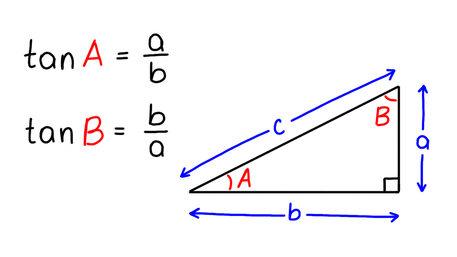VIEW IN FULL SCREEN

# AQA GCSE Maths TangentTangent of an angle is a ratio between the side opposite to the angle and the side adjacent to the angle.Tangent is one of three trigonometric functions of an angle.tan(x) is found by calculating the ratio of two sides of a right-angled triangle, the opposite and the adjacent.The diagram shows a tangent graph.# ✅FULL SCREEN

# ✅